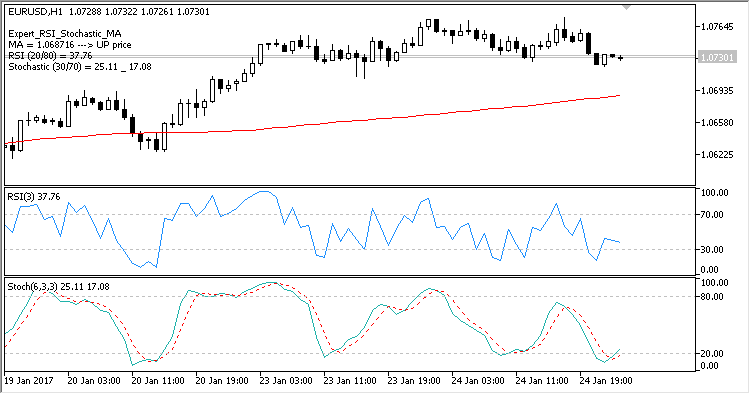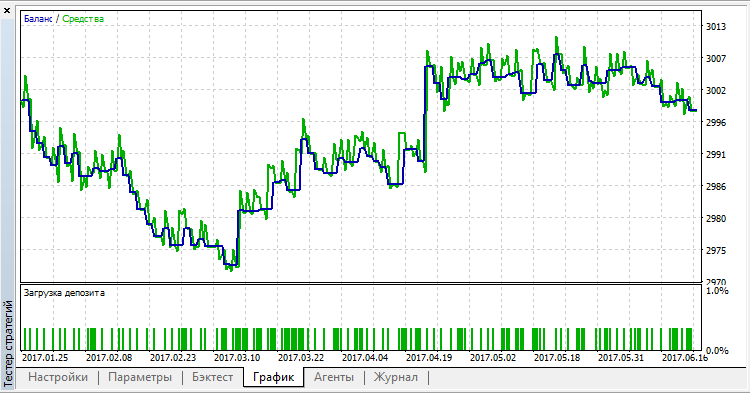# Expert_RSI_Stochastic_MA – expert for MetaTrader 5

• A+

The author of the idea is Oksana Berenko, and the author of the mq5 code is barabashkakvn.

The Expert Advisor uses three indicators: MA(150), RSI(3) with levels 80 and 20, Stochastic(6, 3, 3) with levels 70 and 30. You can change these parameters and set your own values.

1. Trade entry direction is determined based on MA (Moving Average). Only one trade is opened in one direction.

• If Bid > MA, consider the buy direction.
• If Ask < MA, consider the sell direction.

2. Position entry is performed when RSI and Stochastic conditions are met.

• Buy if RSI and Stochastic are below the lower level, i.e. RSI < 20 and Stochastic < 30.
• Sell if RSI and Stochastic are above the upper level, i.e. RSI > 80 and Stochastic > 70.

3. Exit is based on Stochastic.

Exit with profit points.

• (Trailing Stop = 0) If Trailing Stop is set to zero, close a position upon reaching the opposite Stochastic level, if the trade has a certain profit in points.

b) Close BUY if Stochastic > 70 and OpenPrice <= Bid
s) Close SELL if Stochastic < 30 and OpenPrice >= Ask

• (Trailing Stop > 0) If the Trailing Stop value is specified, then once the opposite Stochastic level is reached, the position Stop Loss will be trailed at the opening of each new candlestick, keeping the specified distance form the price. Note that in this case a position can be closed with a loss, since Stop Loss sometimes is not immediately moved to a breakeven level.

Closing with a loss in points.

• (allow Loss = 0) If 'allow Loss' is set to 0, close a position upon reaching the opposite Stochastic level, if the trade has a certain loss in points.

b) Close BUY if Stochastic > 70 and OpenPrice > Bid
s) Close SELL if Stochastic < 30 and OpenPrice < Ask

• (allow Loss > 0) If 'allow Loss' is specified, and we have exited the position entry zone as per Stochastic, while a trade has a loss specified in points or even more, the position is closed.

b) Close BUY if Stochastic > 30 and OpenPrice - Bid >= allow Loss in points
b) Close SELL if Stochastic < 70 and Ask - OpenPrice >= allow Loss in pointsTesting on EURUSD,H1: